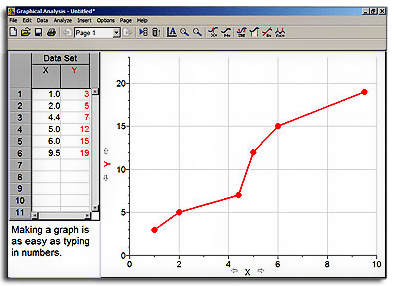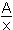# Graphical Analysis

In most cases, line graphs are the most useful for scientists. You will use a program called Graphical Analysis to create line graphs in this course.

First, install the program or decide what you will use.

1. It is suggested you install the Graphical Analysis program. You may need your parents’ help with this. (I was able to install and complete the examples myself, so it is doable.) The directions and link are all on the page above.
2. If you have a tablet you can use: Vernier Graphical Analysis. If the GVL program does not work for you, you can use these: for Mac and for Chrome.
3. However, you could use any graphing program, even Excel, or just your hand and some graph paper.
• Once you are set, scroll down to the “Now You Try It.” (linked in #2)
1. Graph the data and answer the questions without looking at the answers until you answer them. You can see the answers by highlighting them, clicking and dragging, or double clicking over them. They are right next to the word “Answer.”

### Installing Graphical Analysis

Purpose: To install Graphical Analysis (a computer graphing program) on your computersource
Introduction: As you have probably already experienced, graphing data “by hand” can be very tedious and time-consuming. Using a program like Graphical Analysis 3 can make graphing data a quick activity.  Students often expressed that Graphical Analysis is a very student-friendly program. Please email the instructor if you have any questions.

Materials: Graphical Analysis 3

Procedure: You will be using a graphing program for many of your labs in this Chemistry class. This procedure will help you to install Graphical Analysison your computer. Be sure to read and follow the instructions below.

1. Go to the desktop of your computer and create a new folder. You do this by right clicking on your desktop and choosing New Folder. Name the folder “graphing.

2. Click on the file below called Graphical Analysis.zip. You should get a box that asks you if you want to “save the file to disk“. Save the file into the new folder you created called Graphing. This is a zipped file. You will have to unzip it to use the application by following the next steps.

Graphical Analysis.zip

3. Unzip the file

• Go to the folder on your desktop that is labeled “Graphing”.
• Open it and right click on the Graphical Analysis file that is located there.
• Scroll down to “Open with” and choose whatever Zip Utility you have on your computer. Usually WinZip is available.
• When the Zip Utility opens, click on the file, choose extract, and allow it to extract to the “Graphing” folder you created. This will put the application file (unzipped) in the same folder as the zipped file.

When you are ready to use the Graphical Analysis program, just open the Graphing folder on your desktop and double click on the application icon (GA).

If you have any problems installing and running the program, please contact your instructor. You will need the program for the next lesson. Once you have the program running properly, move on to the next lesson where we will learn how to graph using the program.

### Graphical Analysis Tutorial  (Click this link!)

Now you try it

If data points lie roughly along a straight line, the x and y variables have a linear relationship or are directly proportional.  This means as one variable increases, the other does too, in a constant proportion – as x doubles, y doubles; as x triples, y triples; etc.  Directly proportional quantities, x and y, relate to one another through mathematical equations of the form y = mx + b, where m is a constant and b is zero.  The equation for the directly proportional linear relationship is y = kx.  Here m=k and b=0.  For an indirect relationship, one variable increases while the other decreases at a constant rate.  The mathematical expression for an indirect relationship is y = -kx.

If data points lie along a curve that drops from left to right, then the quantities have an inverse relationship or are inversely proportional.  In an inverse relationship, one quantity increases as the other decreases.  The mathematical relationship that expresses an inverse relationship is.  The expression relating gas pressure and volume follows the expression PV=k.  Note that an inverse relationship is nonlinear because the increase of one variable is not accompanied by a constant rate of decrease in the other variable. In summary:

k = the proportionality constant.

If two quantities are directly proportional k = y/x.

If two quantities are inversely proportional k = xy.

Purpose:  to determine the relationship between mass and volume of aluminum; to determine the relationship between the pressure and volume of a gas

Procedure:  Use Graphical Analysis program for entering and analyzing data.

Entering Data:  A Best-Fit Straight Line

1. When the program opens, a data table will be on the left hand side of the screen.  Double click on the x in the data table.  Enter “Volume” for the x axis title and “cubic centimeters” for the units.  Click DONE.

2. Double click on the y in the data table.  Enter “Mass” for the y axis title and “grams” for the units.  Click DONE.

3. Enter the data from the data table below for the mass and volume of aluminum samples. The graph will instantly appear as you enter values.

Volume (cm3) Mass (g)
5.3 14.1
7.5 20.5
10.8 29.0
15.0 39.6
18.4 51.3
20.2 53.7
23.1 64.8

4. After you have entered all the values, click on the graph to select it and then click on the R= button, located on the toolbar, second button from the right side.  This will put a statistics box on the graph showing the slope (m), the y-intercept (b) and the correlation coefficient (ideally 1.00 or -1.00).  This button also draws the best fit straight line for the data points (called a regression line).

5. Double click on the graph to enter a title.  A box will appear where you can type the title “Volume vs. Mass”.  Titles on science graphs are usually in the y vs. x format.  Also on this screen, remove the option of CONNECT POINTS by clicking on the checkmark to deselect it.  This way the graph shows the best fit line for the data points and not a dot-to-dot line.

Creating a Graph for a Best-Fit Curved Line

6. Close, but save, the previous graph and open a new graph and data table.

7. Enter the values for the pressure and volume of a gas as given below and create labels and units for the axes as well as a title for the graph.  Remove CONNECT POINTS as above.

Pressure (atm) Volume (L)
0.100 245
0.200 122
0.400 61.0
0.800 30.4
2.00 12.2
4.00 5.98
8.00 2.92

8.  Notice that the line does not look like a straight fit.  To find the best fit curve line, click on the “f(x)” button on the far right of the tool bar at the top of the screen.

9.  When the next screen opens, a smaller version of your data points will be on the screen with some options at the bottom for the type of curve.  You can click on each one as you move down and then click on TRY FIT at the bottom of the window to see if this curve fits the data points.  For this particular curve, the inverse relationship works best – this is.

10. Save a copy of this graph.

## Questions

1. What is the slope for the first graph?

2. What type of relationship is indicated in Graph 1?

3. Write the equation for the line in the y=mx + b format, substituting the slope for m and zero for b.

Answer: y = 2.786x + 0

4. If the mass of the aluminum sample was 17 g, what volume would this correspond to? Read this from the graph. This is called interpolating data. Finding a data point outside the data range is called extrapolating data.

5. Using the equation from question 3, solve for the volume (x) when the mass (y) is 17 g.

Answer: x = 6.1 (Substitute 17 into the y = mx + b equation.)

Does this answer agree with what you read from the graph?  If it does not, recheck your math or the graph.

6. For Graph #2, what type of relationship is indicated?